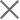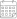Subscribe

### Indexes

Quarter 3-4, 2022 | Energy Market Review30 June 2023

In the third and fourth quarters of 2022, Georgian power plants generated 4,193 mln. and 3,359 mln. kWh of electricity, respectively (Figure 1). This represents a +11.4% and -3.5% change in total generation compared to the corresponding periods of the previous year (in 2021, the total generation in Q3 was 3,766 mln. kWh and 3,479 mln. kWh in Q4). On an annual basis, the increase in generation in the third quarter of 2022 was derived from a 36.4%, 18.5%, and 10.1% increase in thermal, wind, and hydropower generation, respectively. The decrease in production in the fourth quarter is related to the 0.8% and 11.3% decrease in wind and hydropower generation, more than offsetting the 23.3% increase in thermal power generation.

In the third and fourth quarters of 2022, electricity consumption on the local market amounted to 3,434 mln. (Q3) and 3,253 mln. kWh (Q4) (+0.6% and -4.6% compared to the third and fourth quarters of 2021, respectively) (Figure 1). Total power generation exceeded consumption by 759 mln.kWh in the third quarter of the year and by 106 mln.kWh in the fourth quarter. In percentage terms, in Q3 there was a generation surplus amounting to 18.1% of total generation and 3.2% of total generation in Q4, respectively. In 2021, total generation exceeded consumption in the third quarter by 351 mln. kWh, around 9.3% of the total generation, and the difference between total generation and consumption resulted in a surplus in the fourth quarter of 69 mln. kWh, around 2% of the total generation.